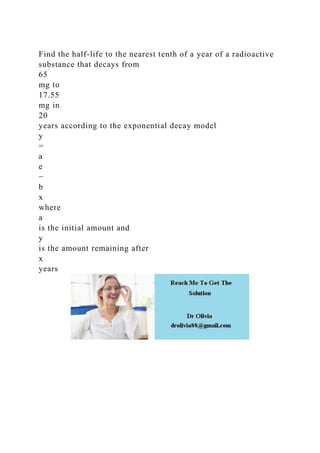Diese Präsentation wurde erfolgreich gemeldet.

# Find the half-life to the nearest tenth of a year of a radioactive s.docx

Anzeige
Anzeige
Anzeige
Anzeige
Anzeige
Anzeige
Anzeige
Anzeige
Anzeige
Anzeige
Anzeige×

1 von 1 Anzeige

# Find the half-life to the nearest tenth of a year of a radioactive s.docx

Find the half-life to the nearest tenth of a year of a radioactive substance that decays from
65
mg to
17.55
mg in
20
years according to the exponential decay model
y
=
a
e

b
x
where
a
is the initial amount and
y
is the amount remaining after
x
years
.

Find the half-life to the nearest tenth of a year of a radioactive substance that decays from
65
mg to
17.55
mg in
20
years according to the exponential decay model
y
=
a
e

b
x
where
a
is the initial amount and
y
is the amount remaining after
x
years
.

Anzeige
Anzeige

### Find the half-life to the nearest tenth of a year of a radioactive s.docx

1. 1. Find the half-life to the nearest tenth of a year of a radioactive substance that decays from 65 mg to 17.55 mg in 20 years according to the exponential decay model y = a e − b x where a is the initial amount and y is the amount remaining after x years